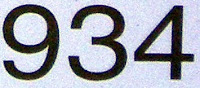## Monday, March 28, 2011

### 934

934 = 2 x 467.

934 has a fifth root that starts 3.25252225 . . .

934 is a number of the form n2 + n + 4, for n = 30.

934 is the number of positions that the 3 x 3 x 3 Rubik's cube can be in after exactly five moves (under the full group of order 48 of the cube). This is the number of positions that can be reached in five moves, but which cannot be reached in fewer than five moves.

934 is the sixteenth Fibonacci number minus the sixteenth prime number: 934 = 987 - 53.The Porsche 934, introduced in 1976, was a racing version of the Porsche 911 Turbo.